# Economics Study Set 17

## Quiz 7 : Production, Inputs, and Cost: Building Blocks for Supply AnalysisStudy FlashcardsLooking for Economics Homework Help?

## Quiz 7 :Production, Inputs, and Cost: Building Blocks for Supply Analysis

Question TypeA firm hires two workers and rents 15 acres of land for a season. It produces 150,000 bushels of crop. If it had doubled its land and labor, production would have been 325,000 bushels. Does it have constant, decreasing, or increasing returns to scale
Free
Essay

A firm will have increasing returns to scale if the output increases more than the proportionate increase in the inputs. If the change in the firm's input leads to more production than the changed input, it is increasing returns to scale. If the output is less than the input in the changed input of the firm, it is decreasing returns to scale.
Initially firm produces 150,000 bushels of crop and after doubling the inputs the firm produced 325,000 bushels of crop. The increase in the output is more than that of proportionate increase in the inputs.
Therefore, firm has an increasing return to scale considering an increase in the output at increasing rate.

Tags
Choose question tagWith the following data, calculate the firm's AVC and MVC and draw the graphs for TVC, AVC, and MVC. Why is MVC the same as MC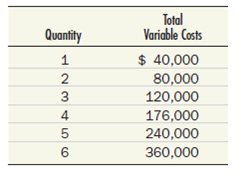Free
Essay

Average variable cost is calculated by considering the total variable cost and the quantity. Marginal variable cost is equal to change in total variable cost divided by change in the quantity. The following is a measurement of average variable cost and marginal variable cost.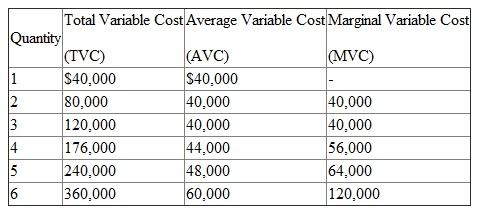In the above table, one has calculated the AVC and MVC with the help of given information. Both AVC and MVC increase as the quantity of production increases.
The following is graphical representation of the average variable cost, total variable cost, and marginal revenue cost curves.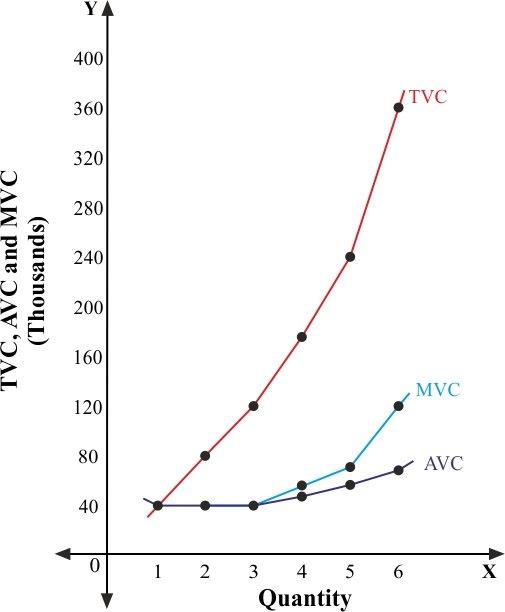In the above diagram, one has taken quantity on x-axis and TVC, AVC, and MVC on the y-axis. TVC slopes upward to the right because variable cost increases as output increases. AVC and MVC are constant at initial stage and then both slopes upward.
Marginal cost is equal to marginal fixed cost plus marginal average cost. Marginal fixed cost are always zero, therefore the marginal variable cost equals marginal cost. Symbolically,Therefore, marginal cost is equal to marginal variable cost.

Tags
Choose question tagSuppose that wages are $20,000 per season per person and land rent per acre is$3,000. Calculate the average cost of 150,000 bushels and the average cost of 325,000 bushels, using the figures in Test Yourself Question 6. (Note that average costs increase when output increases.) What connection do these figures have with the firm's returns to scale
Free
Essay

Average cost is equal to total cost divided by the total quantity of the firm. Total wage of two labors are $40,000 (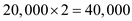), total rent is$45,000 (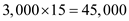Thus, the total cost is $85,000 (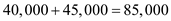). The total quantity is 150,000 bushels. Thus, the average cost of 150,000 bushels is$0.56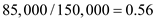).
If the input doubles, then total wage of two labors are $80,000 (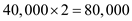), total rent is$90,000 (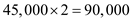).
The total cost is $170,000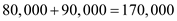). The total quantity is 325,000 bushels. Thus, the average cost of 325,000 bushels is$0.52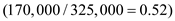this lower average cost indicates the increasing returns to scale.

Tags
Choose question tagIf a firm's commitments in 2011 include machinery that will need replacement in five years, a factory building rented for 12 years, and a three-year union contract specifying how many workers it must employ, when, from its point of view in 2011, does the firm's long run begin
Essay
Tags
Choose question tagLabor costs $12 per hour. Nine workers produce 180 bushels of product per hour, whereas 10 workers produce 196 bushels. Land rents for$1,200 per acre per year. With 10 acres worked by nine workers, the marginal physical product of an acre of land is 1,400 bushels per year. Does the farmer minimize costs by hiring nine workers and renting 10 acres of land If not, which input should he use in larger relative quantity
Essay
Tags
Choose question tagA firm's total fixed cost is $360,000. Construct a table of its total and average fixed costs for output levels varying from zero to six units. Draw the corresponding TFC and AFC curves. Essay Answer: Tags Choose question tagA firm experiences a sudden increase in the demand for its product. In the short run, it must operate longer hours and pay higher overtime wage rates to satisfy this new demand. In the long run, the firm can install more machines instead of operating fewer machines for longer hours. Which do you think will be lower, the short-run or the long-run average cost of the increased output How is your answer affected by the fact that the long-run average cost includes the new machines the firm buys, whereas the short-run average cost includes no machine purchases Essay Answer: Tags Choose question tagFrom the data in Test Yourself Questions 1 and 2, calculate TC and AC for each of the output levels from one to six units and draw the two graphs. Essay Answer: Tags Choose question tagRemember Al, the builder of inexpensive garages Suppose that his total costs increase by$5,000 per year at every input level. Modify Table 2 to show how this change affects his total and average costs.
Essay
Tags
Choose question tagNaomi has stockpiled a great deal of chicken feed. Suppose now that she buys more chicks, but not more chicken feed, and divides the feed she has evenly among the larger number of chickens. What is likely to happen to the marginal physical product of feed What, therefore, is the role of input proportions in the determination of marginal physical product
EssayIf the marginal revenue product of a gallon of oil used as input by a firm is $2.20 and the price of oil is$2.07 per gallon, what can the firm do to increase its profits